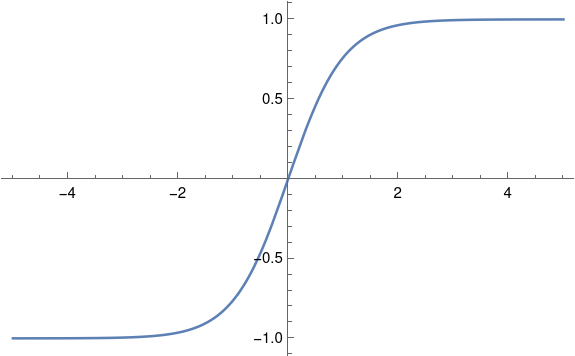# Smoothed step function

I mentioned smoothed step functions in the previous post. What would you do if you needed to concretely use a smoothed step function and not just know that one exists?

We’ll look at smoothed versions of the signum function

sgn(x) = x / |x|

which equals -1 for negative x and +1 for positive x. We could just as easily looked at the Heaviside function H(x), which is 0 for negative x and 1 for positive x, because

H(x) = (1 + sgn(x))/2

and so if we can approximate signum we can approximate the Heaviside function.

We want our approximation of sgn(x) to be -1 for x < –c and +1 for x > c. We also want our approximation to be smooth everywhere and monotone increasing on [-c, c].

## Approximate solution

Things are much simpler if we’re willing to tolerate a small error in the flat regions. Then we could use

f(x; k) = 2 arctan(kx)/π

or

g(x; k) = tanh(kx).

The parameter k controls how sharply the function rises in the transition interval [-c, c], with larger values of k corresponding to steeper transitions. You could solve for the value of k that gives you the desired slope at 0, or that meets your error tolerance at ±c.

Here’s a plot of hyperbolic tangent, i.e g(x; 1).## Solving for the parameter k

The derivative of f(x; k) at zero is 2k/π. So if your desired slope is m, then

k = πm/2.

The derivative of g(x; k) at zero is simply k, so set k = m.

If you want the value of your approximation to be between 1 – ε and 1 for x > c, and by symmetry between -1 and -1 + ε for x < –c, you can solve

f(c, k) = 1 – ε

to get

k = tan(π(1 – ε)/2)/c

or solve

g(c, k) = 1 – ε

to get

k =  arctanh(1 – ε)/c.

If you don’t have a way to directly compute arctanh, you can use

arctanh(x) = log( (1 + x) √(1/(1 – x²)) ).

The equation above and similar equations can be found here.

## Exact solution

It would be unusual to need an exact explicit solution. In applications, you need to be explicit but not exact. In theoretical work, you often need to be exact but not explicit. I will outline a way to create an smoothed step function that is n times differentiable.

Let f(x; a) be the PDF of a Beta(a, a) random variable and let F(x; a) be the corresponding CDF. Then F(x; a) = 0 for x < 0 and F(x; a) = 1 for x > 1.

If a is greater than n then F is n-times differentiable at 0, and by symmetry the same holds at 1. For integer a, F(x; a) is a polynomial over [0, 1], but a needs to be large enough that the first n derivatives are all 0 at the ends.

The larger a is, the steeper F is in the middle. F has a transition zone over [0, 1], but you can shift and scale F to transition over [-c, c].